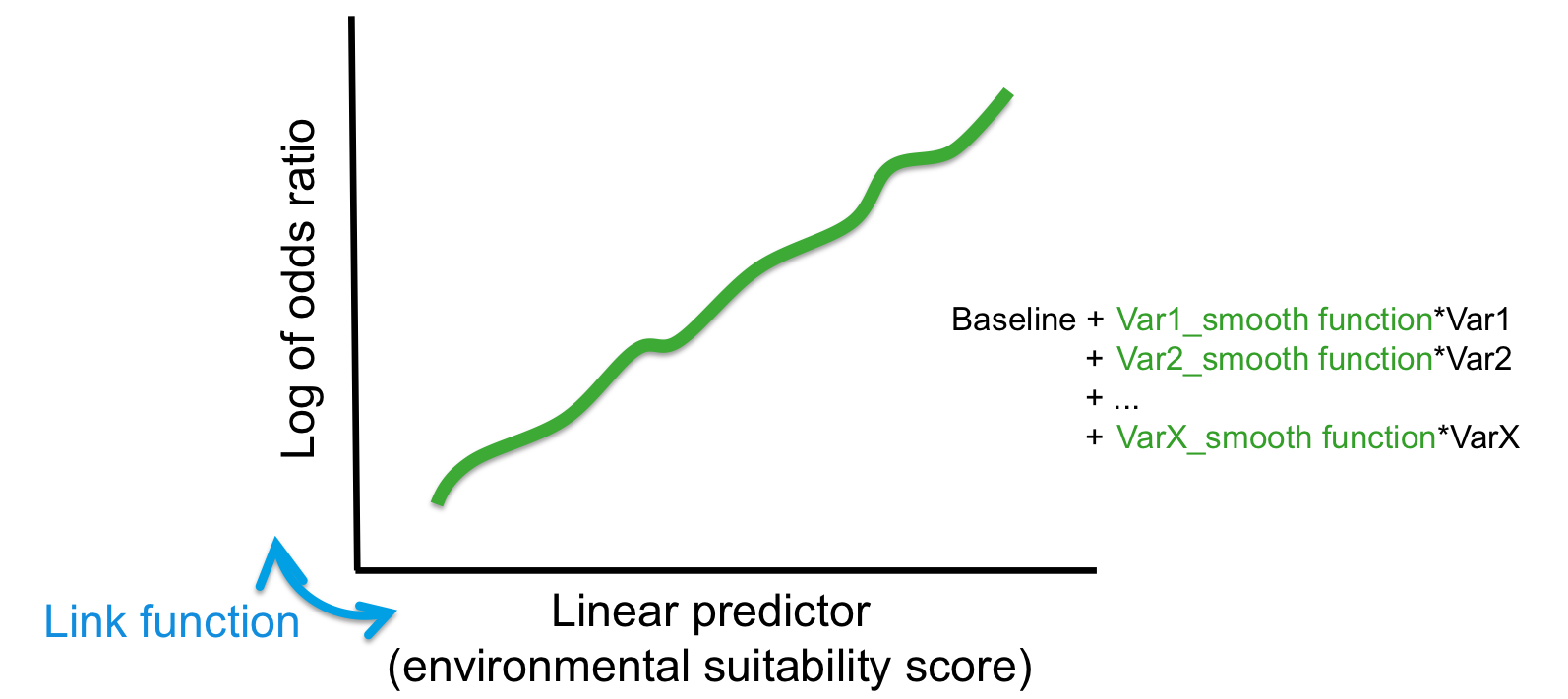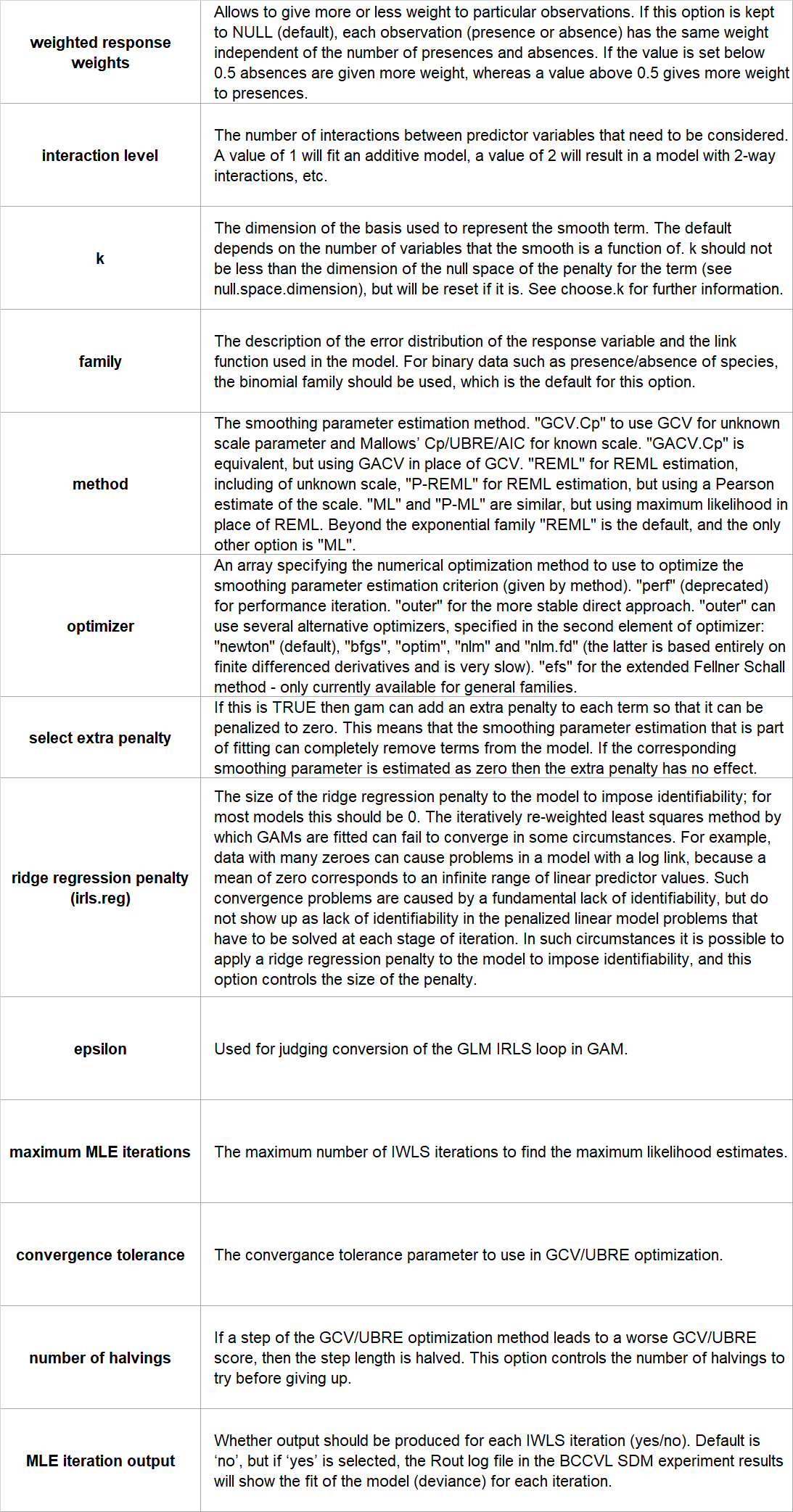## Introduction

Generalized Additive Models (GAMs) are an extension of Generalized Linear Models (GLMs) in such a way that predictor variables can be modeled non-parametrically in addition to linear and polynomial terms for other predictors. Therefore, GAMs are useful when the relationship between the variables are expected to be of a more complex form, not easily fitted by standard linear or non-linear models, or where there is no a priori reason for using a particular model.

Like GLMs, GAMs have three important components:

1. the probability distribution of the response variable
2. the linear predictor (LP), which is a combination of all predictor variables and represents an overall score for the environmental suitability.
3. the link function that describes how the mean of the response depends on the linear predictor.

However, in GAMs the coefficients of the predictor variables in the linear predictor are replaced by a smoothing function. The model fits a smooth curve to each predictor variable and then combines the results additively. The GAM algorithm in BCCVL uses a cubic spline smoother.The estimation of the values of the variable coefficients is obtained by maximum likelihood estimation (MLE), which maximizes the agreement of the predicted species occurrences with the observed data. In other words, MLE finds the values of the coefficients that result in a model under which you would be most likely to get the observed results. As for GLM models, GAM uses the iteratively reweighted least squares (IWLS) method for MLE.

• Able to deal with non-linear and non-monotonic relationships between the response and the predictor variables.
• Able to deal with categorical predictors.

### Limitations

• More susceptible to overfitting. To avoid this, it is good practice to compare the model fit of a GLM with the fit of a GAM and evaluate whether the added complexity of GAMs is necessary in order to obtain a satisfactory fit to the data. If the fit of a GLM and GAM is comparable, it is advised to use a GLM model.
• Less easy to interpret compared to GLMs.

### Assumptions

No assumptions are made about the distributions of the environmental variables. However, they should not be highly correlated with one another because this could cause problems with the estimation.

Yes.

### Configuration options

BCCVL uses the ‘gam’ function in the ‘mgcv’ package, implemented in biomod2. The user can set the following configuration options:### References

• Elith J, Graham CH, Anderson RP et al. (2006) Novel methods improve prediction of species’ distributions from occurrence data. Ecography, 29(2), 129-151.
• Franklin J (2010) Mapping species distributions: spatial inference and prediction. Cambridge University Press.
• Guisan A, Edwards TC, Hastie T (2002) Generalized linear and generalized additive models in studies of species distributions: setting the scene. Ecological modelling, 157(2), 89-100.
• Hastie T, Tibshirani R, Friedman J (2009) The elements of statistical learning: data mining, inference and prediction. 2nd edition, Springer.